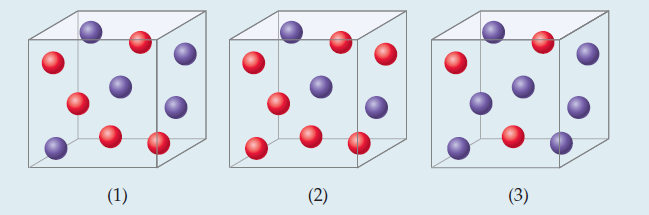×
Get Full Access to Chemistry: The Central Science - 12 Edition - Chapter 14 - Problem 4pe
Get Full Access to Chemistry: The Central Science - 12 Edition - Chapter 14 - Problem 4pe

×

# Relating a Rate Law to the Effect of Concentration onISBN: 9780321696724 27

## Solution for problem 4PE Chapter 14

Chemistry: The Central Science | 12th Edition

• Textbook Solutions
• 2901 Step-by-step solutions solved by professors and subject experts
• Get 24/7 help from StudySoup virtual teaching assistantsChemistry: The Central Science | 12th Edition

4 5 1 356 Reviews
28
2
Problem 4PE

Consider a reaction A + B → C for which rate = k[A][B]2. Each of the following boxes represents a reaction mixture in which A is shown as red spheres and B as purple ones. Rank these mixtures in order of increasing rate of reaction.Assuming that rate = k[A][B], rank the mixtures represented in this Sample Exercise in order of increasing rate.

Step-by-Step Solution:
Step 1 of 3

NEWTON’S LAWS OF MOTION 1. Objects at rest stay at rest and objects in motion stay in motion unless an outside force acts a. force changes motion b. rest feels the same as uniform motion i. you only feel changes in motion c. there’s no such thing as centrifugal force 2. Acceleration (change in motion) is directly proportional to applied force and inversely proportional to inertia (mass) a. a = f/m i. usually written as F = ma b. weight is not the same as mass c. mass is the property of an object (its inertia) d. weight = force of gravitation ON an object 3. Every action has an equal and opposite reaction a. forces always come in pairs i.

Step 2 of 3

Step 3 of 3

##### ISBN: 9780321696724

Unlock Textbook Solution